1. Fermat's point of the triangle

On the sides of an a triangle ABC with all angles less that 2π/3 construct equilateral triangles BCK, CAL, ABM.
Then
a) Lines AK, BL, CM are all equal.
b) All these lines pass through a point F.
c) All the angles formed at F by these lines are 60 degrees.
d) The centers of the equilateral triangles form again an equilateral triangle UVW (see Napoleon.html ).
e) The point F minimizes the sum of distances f(P) = PA+PB+PC.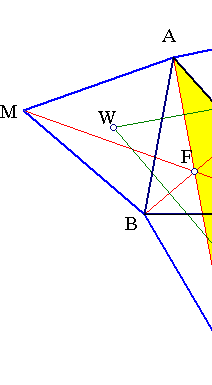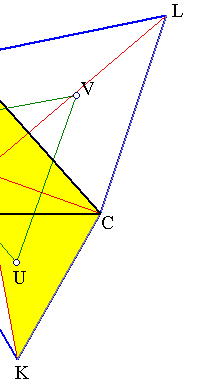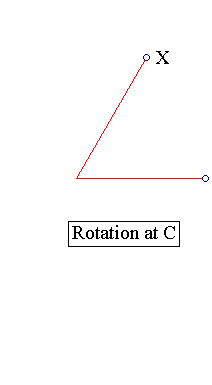For (a):  compare triangles AKC, LBC and show them equal. The second results from the first by turningit by an
angle of π/3 about C. This implies also that the angle of AK to BL is π/3. From the equality ofangles AFL and
ACL follows that AFCL is cyclic, this implying that KFC is also π/3, thus F is distinguished on BL by its
property to view BC under the angle BFC which is 2π/3. This implies easily all propertiesup to (d).
For (e): Take a point P, join with A, B and C. Turn the triangle APC about A by an angle of 60 degrees (figure
below). You want to minimize BP+AP+CP = BP+PQ+QL. But this is always shorter than the segment BL.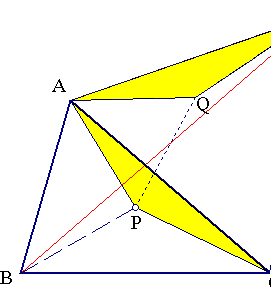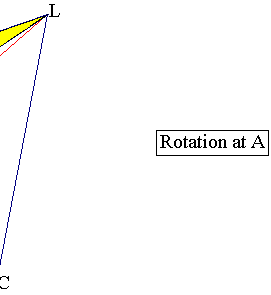2. The case A > 2π/3

The results are true with some minor changes also in the case one angle of  the triangle is > 120 degrees. Lines
AK, BL, CM are again equal but this time intersect at a point F on the extension of AK, for A>2π/3.
Also point F in this case is viewing BC under an angle of measure 2π/3, whereas the other sides are seen
under an angle of &p;i/3.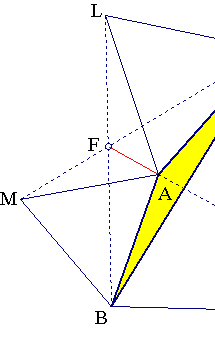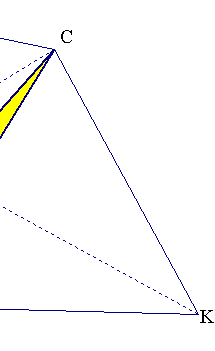3. The other Fermat point

The construction of the equilaterals can be carried out inwards, creating a second configuration analogous to
the more usually drawn. Again segments {AK, BL, CM} have equal lengths and pass through the same point,
which is viewing the sides under π/3 or 2π/3 angles. The point F' thus defined is the second Fermat
point of the triangle.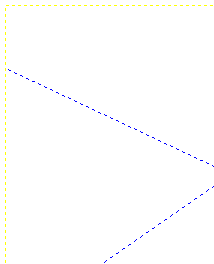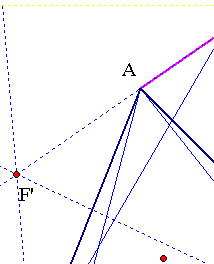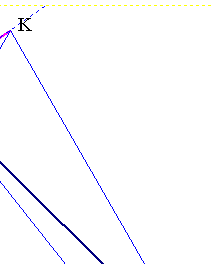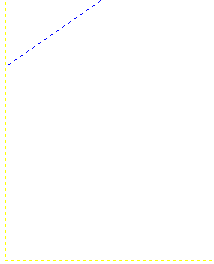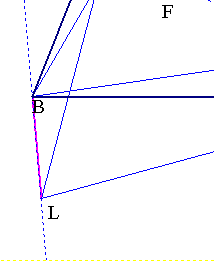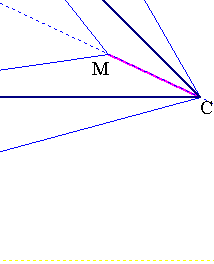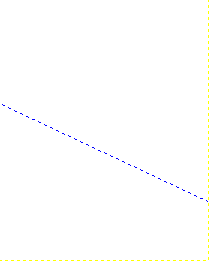4. Trilinear coordinates

The trilinear coordinates (x:y:z) of the Fermat's point F are easily calculated using the properties of the figure
proven above (see Trilinears.html ).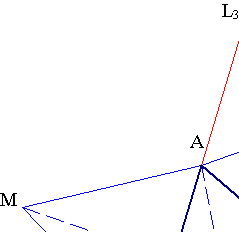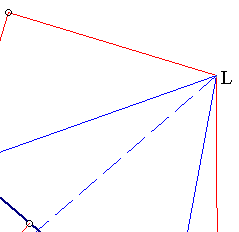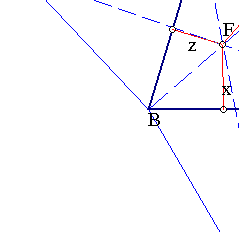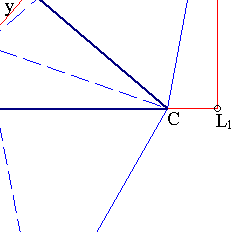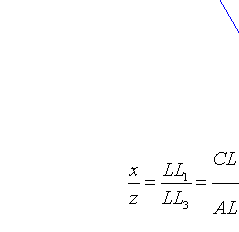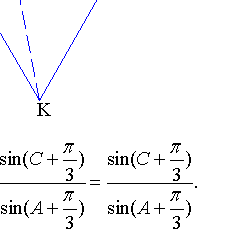Last cancelation of factors due to the equilateral ACL. Hence the trilinears of points F, and, working analogously
for F', are given by the ratios: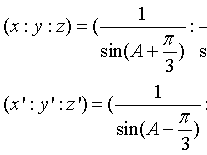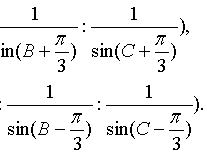Thus, it is seen that {F,F'} are isogonal conjugate (see Isogonal_Conjugation.html ) to the isodynamic points {J, J'} of
the triangle (see Isodynamic.html and for the calculation of trilinears of {J, J'} see ApolloniusCircles.html ).